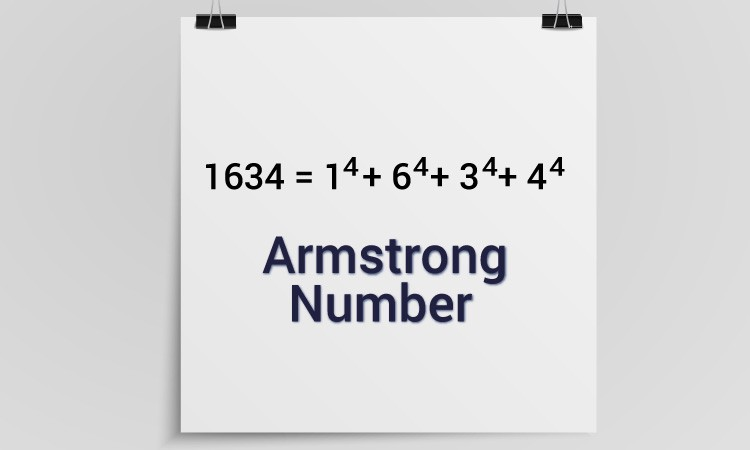# Armstrong Number Check of a given number in input using Python3

## ARMSTRONG NUMBER CHECK in Python

Armstrong Number Check: The Number is known as Armstrong Number if and only if the following condition satisfies.

Sum of: ((each digit of the Number)^(Number of Digits of the Number)) = Number

i.e., To be an Armstrong Number, the sum of the digits, to the power of the number of digits, should be equal to the number itself. These are the examples: 153, 1634 etc.The following code snippet is the program to detect Armstrong Number and is written in Python3:

### Python program to check if a given number is Armstrong or not

```n=int(input("Input a number of two or more digits:"))
t=n
check=s=count=0
num=[]
while(check==0):
i=n%10      #take out the last digit
n=(n-i)/10  #update the original input by removing the last digit
n=round(n)
num.append(i)   #list every digit
count=count+1
if(n<10):
num.append(n)
break
for i in range(len(num)):
s+=num[i]**(count+1) # (sum of each digit)^(number of digits in the input number)
print("Sum is:",s)
print("Number of digits is:",count+1)
if(s==t):
print("The entered number is an Armstrong Number.")
else:
print("The entered number is not an Armstrong Number.")```

OUTPUT 1:

```Input a number of two or more digits:1645
Sum is: 2178
Number of digits is: 4
The entered number is not an Armstrong Number.```

OUTPUT 2:

```Input a number of two or more digits:153
Sum is: 153
Number of digits is: 3
The entered number is an Armstrong Number.```